Updating search results...

# 11 Results

View
Selected filters:
• diameterRating
0.0 stars

Students compare the relationships between radius, diameter, circumference, and area by manipulating a circle and increasing or decreasing its size in this interactive from Illuminations. It also provides a problems section where students determine the answer to each question using the applet, a calculator, or paper and pencil. They must also indicate the proper units!

Subject:
Geometry
Mathematics
Material Type:
Activity/Lab
Interactive
Provider:
National Council of Teachers of Mathematics
Author:
Illuminations
02/26/2019Rating
0.0 stars

The Greek mathematician Archimedes approximated pi by inscribing and circumscribing polygons about a circle and calculating their perimeters. Similarly, the value of pi can be approximated by calculating the areas of inscribed and circumscribed polygons. This activity allows for the investigation and comparison of both methods.

Subject:
Mathematics
Material Type:
Activity/Lab
Interactive
Provider:
National Council of Teachers of Mathematics
Author:
Illuminations
02/26/2019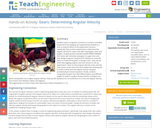Educational Use
Rating
0.0 stars

Students work as engineers and learn to conduct controlled experiments by changing one experimental variable at a time to study its effect on the experiment outcome. Specifically, they conduct experiments to determine the angular velocity for a gear train with varying gear ratios and lengths. Student groups assemble LEGO MINDSTORMS(TM) NXT robots with variously sized gears in a gear train and then design programs using the NXT software to cause the motor to rotate all the gears in the gear train. They use the LEGO data logging program and light sensors to set up experiments. They run the program with the motor and the light sensor at the same time and analyze the resulting plot in order to determine the angular velocity using the provided physics-based equations. Finally, students manipulate the gear train with different gears and different lengths in order to analyze all these factors and figure out which manipulation has a higher angular velocity. They use the equations for circumference of a circle and angular velocity; and convert units between radians and degrees.

Subject:
Applied Science
Engineering
Material Type:
Activity/Lab
Provider:
TeachEngineering
Provider Set:
TeachEngineering
Author:
James Cox
Jasmin Mejias
Jennifer S. Haghpanah
Leonarda Huertas
Mihai Pruna
09/18/2014Conditional Remix & Share Permitted
CC BY-NC
Rating
0.0 stars

Four full-year digital course, built from the ground up and fully-aligned to the Common Core State Standards, for 7th grade Mathematics. Created using research-based approaches to teaching and learning, the Open Access Common Core Course for Mathematics&nbsp;is designed with student-centered learning in mind, including activities for students to develop valuable 21st century skills and academic mindset.

Subject:
Mathematics
Material Type:
Full Course
Provider:
Pearson
11/02/2020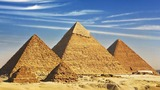Conditional Remix & Share Permitted
CC BY-NC
Rating
0.0 stars

Zooming In On Figures

Unit Overview

Type of Unit: Concept; Project

Length of Unit: 18 days and 5 days for project

Prior Knowledge

Students should be able to:

Find the area of triangles and special quadrilaterals.
Use nets composed of triangles and rectangles in order to find the surface area of solids.
Find the volume of right rectangular prisms.
Solve proportions.

Lesson Flow

After an initial exploratory lesson that gets students thinking in general about geometry and its application in real-world contexts, the unit is divided into two concept development sections: the first focuses on two-dimensional (2-D) figures and measures, and the second looks at three-dimensional (3-D) figures and measures.
The first set of conceptual lessons looks at 2-D figures and area and length calculations. Students explore finding the area of polygons by deconstructing them into known figures. This exploration will lead to looking at regular polygons and deriving a general formula. The general formula for polygons leads to the formula for the area of a circle. Students will also investigate the ratio of circumference to diameter ( pi ). All of this will be applied toward looking at scale and the way that length and area are affected. All the lessons noted above will feature examples of real-world contexts.
The second set of conceptual development lessons focuses on 3-D figures and surface area and volume calculations. Students will revisit nets to arrive at a general formula for finding the surface area of any right prism. Students will extend their knowledge of area of polygons to surface area calculations as well as a general formula for the volume of any right prism. Students will explore the 3-D surface that results from a plane slicing through a rectangular prism or pyramid. Students will also explore 3-D figures composed of cubes, finding the surface area and volume by looking at 3-D views.
The unit ends with a unit examination and project presentations.

Subject:
Geometry
Mathematics
Provider:
PearsonConditional Remix & Share Permitted
CC BY-NC
Rating
0.0 stars

Students will measure the circumference and diameter of round things in the classroom and discover the ratio pi. They will see that the ratio of a circle's circumference to its diameter can be used to solve for the circumference when the diameter is known.Key ConceptsStudents have seen circles before, but have not analyzed the relationships between parts of a circle. The ratio of the circumference to the diameter is pi, about 3.14 or about 227. Students see that all of the objects they measure have this ratio (or close, depending on accuracy) and that the ratio is true for all circles. Students also see that the ratio can be used to solve for the circumference of a circle if the diameter (or radius) is known.GoalsMeasure round things looking for similarities.Find the ratio of the circumference to the diameter of those round things.Find a formula to find the circumference of a circle.SWD: Make sure students understand these domain-specific terms:It may be helpful to preteach these terms to students with special needs. If possible, reinforce the definitions of these terms with visual supports (diagrams).ELL: As new vocabulary is introduced, be sure to repeat it several times and to allow students to repeat after you as needed. Write the new words as they are introduced and allow enough time for ELLs to check their dictionaries or briefly consult with another student who shares the same primary language if they wish.ratiocircumferencecirclediameterscatter plot

Subject:
Geometry
Material Type:
Lesson Plan
Author:
Pearson
11/02/2020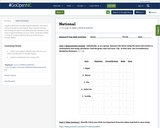Conditional Remix & Share Permitted
CC BY-NC
Rating
0.0 stars

Students will practice measuring the diameter and radius of food themed circular objects. Next, they will discover that finding the ratio of circumference and diameter is the value of approximately 3.14 or pi.
Then, using the number 3.14 for Pi, students will find the area of the various circular objects.

Subject:
Algebra
Geometry
Math 1
Mathematics
Material Type:
Activity/Lab
Homework/Assignment
07/10/2020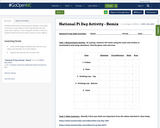Conditional Remix & Share Permitted
CC BY-NC
Rating
0.0 stars

Students will practice measuring the diameter and radius of various circular objects. Next, they will discover the ratio of pi to about 3.
Then, using the number 3.14 for Pi, students will find the area of the various circular objects.

Subject:
Algebra
Geometry
Math 1
Mathematics
Material Type:
Activity/Lab
Homework/Assignment
11/06/2019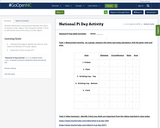Conditional Remix & Share Permitted
CC BY-NC
Rating
0.0 stars

Students will practice measuring the diameter and radius of various circular objects. Then using the number 3.14 for Pi, will find the area of the various circular objects.

Subject:
Algebra
Geometry
Math 1
Mathematics
Material Type:
Activity/Lab
Homework/Assignment
11/06/2019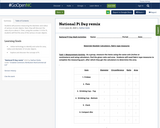Conditional Remix & Share Permitted
CC BY-NC
Rating
0.0 stars

Students will practice measuring the diameter and radius of various circular objects. Next, they will discover the ratio of pi to about 3.
Then, using the number 3.14 for Pi, students will find the area of the various circular objects.

Subject:
Algebra
Geometry
Math 1
Mathematics
Material Type:
Activity/Lab
Homework/Assignment
07/07/2020Rating
0.0 stars

In this video segment from Cyberchase, the CyberSquad is trapped in one of the Spheres of Fears, and they are trying to get through a gate to escape. The key to the gate is locked inside a can, and in order to access the key they must attach the correct label to the can. After guessing incorrectly on the first try, the CyberSquad decides to take some measurements to find the correct label. Using two circles nearby, they compare the diameter of each circle to its circumference. They realize there is a special relationship between the circumference of a circle and the length of its diameter. Teaching tips are also provided that discuss frame, focus and follow-up suggestions for using this video in a math lesson.

Subject:
Geometry
Mathematics
Material Type:
Activity/Lab
Presentation
Provider:
WGBH - Teachers' Domain
Author:
WNET.org